powered by FreeFind

Matrix Row Operations: Examples (page 2 of 2)In practice, the most common procedure is a combination of row multiplication and row addition. Thinking back to solving two-equation linear systems by addition, you most often had to multiply one row by some number before you added it to the other row. For instance, given: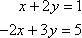...you would multiply the first row by 2 before adding it to the second row: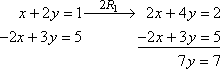Using matrices, the above system looks like this: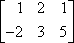Grab some scratch paper for the row calculation: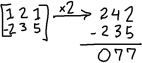...and do the row operation: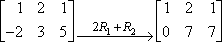The "2R1 + R2" means "I multiplied row one by 2, and then added the result to row two". Note that row one itself is actually unchanged, so it is copied over to the new matrix. Only row two was actually adjusted.   Copyright © Elizabeth Stapel 2003-2011 All Rights Reserved

By the way, for doing operations like this, you'll probably want to use a lot of scratch paper, so you can be careful with your calculations. Or else you'll want to figure out how to have your graphing calculator do the messy parts. For instance, my calculator can do the "2R1 + R2" operation like this: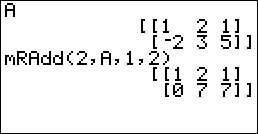Check your manual for the instructions for your model of calculator.

Returning to that last matrix above, this is what the full calculation would look like:

 Hand-in work: Scratch-work:Divide the second row by 7: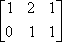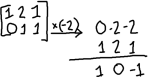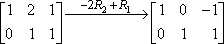Remember to do the scratch-work on scratch paper, not in the margins of your homework. You would hand in the following: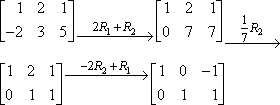The above matrix calculations correspond to solving the linear system "x + 2y = 1, 2x + 3y = 5" to get the solution "x = 1, y = 1".

It's fairly simple to learn the three matrix row operations, but actually doing the operations can be frustrating. It is amazingly easy to make little arithmetic errors that mess up all your calculations. So do your work very clearly, plainly denoting the row operations you are doing. The neater you are, the more likely you'll be to get the right answer in the end, but even if you get a wrong answer, neat work is a lot easier to check.

If you are required to do the work by hand but you have a graphing calculator, ask your instructor if it's okay to do the operations in the calculator (instead of on scratch paper), just writing down the steps you took and copying down the results, because this can really cut down on the errors.

Matrices are quite powerful and versatile, but  good golly!  are they annoying to do by hand!

<< Previous  Top  |  1 | 2  |  Return to Index

 Cite this article as: Stapel, Elizabeth. "Matrix Row Operations: Examples." Purplemath. Available from     https://www.purplemath.com/modules/mtrxrows2.htm. Accessed [Date] [Month] 2016

Reviews of
Internet Sites:
Free Help
Practice
Et Cetera

Study Skills Survey

Tutoring from Purplemath
Find a local math tutor